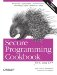# Performing Date and Time Arithmetic

Problem

You want to know the amount of time elapsed between two date/time points.

Solution

If both date/time points falls between the years of 1970 and 2038, you can use a time_t type and the difftime function from the header. Example 5-6 shows how to compute the number of days elapsed between two dates.

Example 5-6. Date and time arithmetic with time_t

```#include
#include
#include

using namespace std;

time_t dateToTimeT(int month, int day, int year) {
// january 5, 2000 is passed as (1, 5, 2000)
tm tmp = tm( );
tmp.tm_mday = day;
tmp.tm_mon = month - 1;
tmp.tm_year = year - 1900;
return mktime(&tmp);
}

return time_t(-1);
}

time_t now( ) {
return time(0);
}

int main( ) {
time_t date1 = dateToTimeT(1,1,2000);
time_t date2 = dateToTimeT(1,1,2001);

cerr << "unable to create a time_t struct" << endl;
return EXIT_FAILURE;
}
double sec = difftime(date2, date1);
long days = static_cast(sec / (60 * 60 * 24));
cout << "the number of days between Jan 1, 2000, and Jan 1, 2001, is ";
cout << days << endl;
return EXIT_SUCCESS;
}```

The program in Example 5-6 should output :

`the number of days between Jan 1, 2000, and Jan 1, 2001, is 366`

Notice that the year 2000 is a leap year because even though it is divisible by 100; it is also divisible by 400, thus it has 366 days.

Discussion

The time_t type is an implementation defined arithmetic type. This means it is either an integer or floating-point type, and thus supports the basic arithmetic operations. You can add, subtract, divide, multiply, and so forth. To compute the distance between two time_t values to seconds, you need to use the difftime function. Do not assume that time_t itself counts seconds, even if it is true. Many C++ implementations may very well quietly change it to count fractions of a second in the near future (this is one reason why difftime returns a double).

If the limitations of time_t are too restricting then you will probably want instead to use the various classes from the Boost date_time library to compute time intervals. Example 5-7 shows how to use the Boost classes to calculate the number of days in the 20th and the 21st centuries.

Example 5-7. Date and time arithmetic with date_duration

```#include
#include

using namespace std;
using namespace boost::gregorian;

int main( )
{
date_duration dd = date(2000, 1, 1) - date(1900, 1, 1);
cout << "The twentieth century had " << dd.days( ) << " days" << endl;
dd = date(2100, 1, 1) - date(2000, 1, 1);
cout << "The twenty-first century will have " << dd.days( ) << " days" << endl;
}```

The program in Example 5-7 outputs:

```The twentieth century had 36524 days
The twenty-first century will have 36525 days```Secure Programming Cookbook for C and C++: Recipes for Cryptography, Authentication, Input Validation & More
ISBN: 0596003943
EAN: 2147483647
Year: 2006
Pages: 241